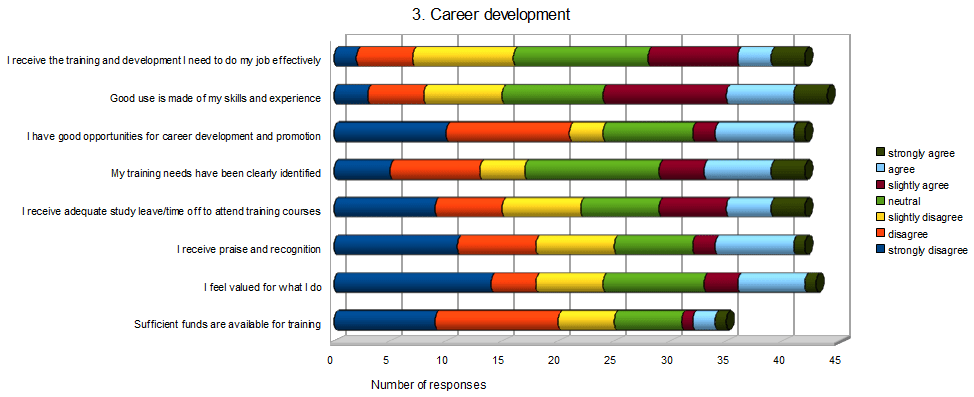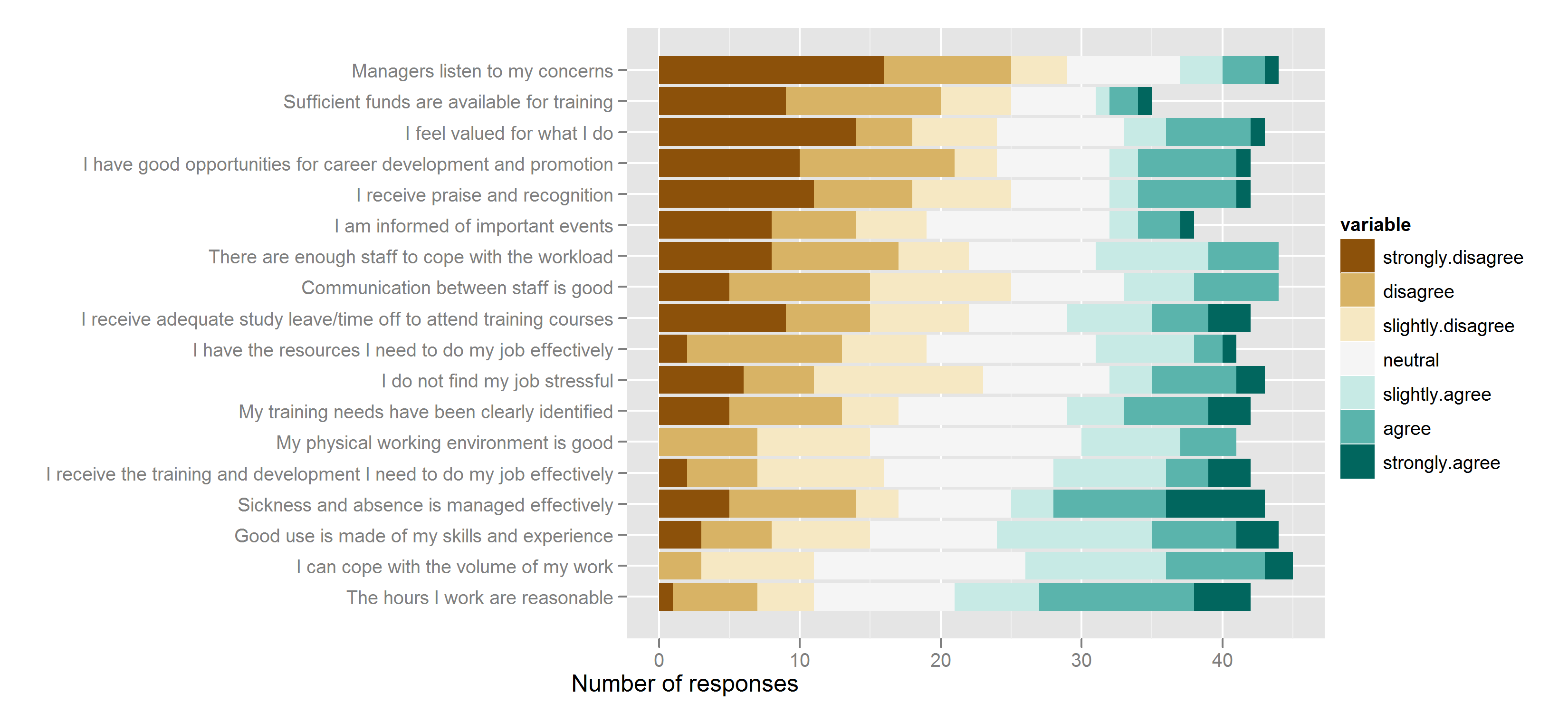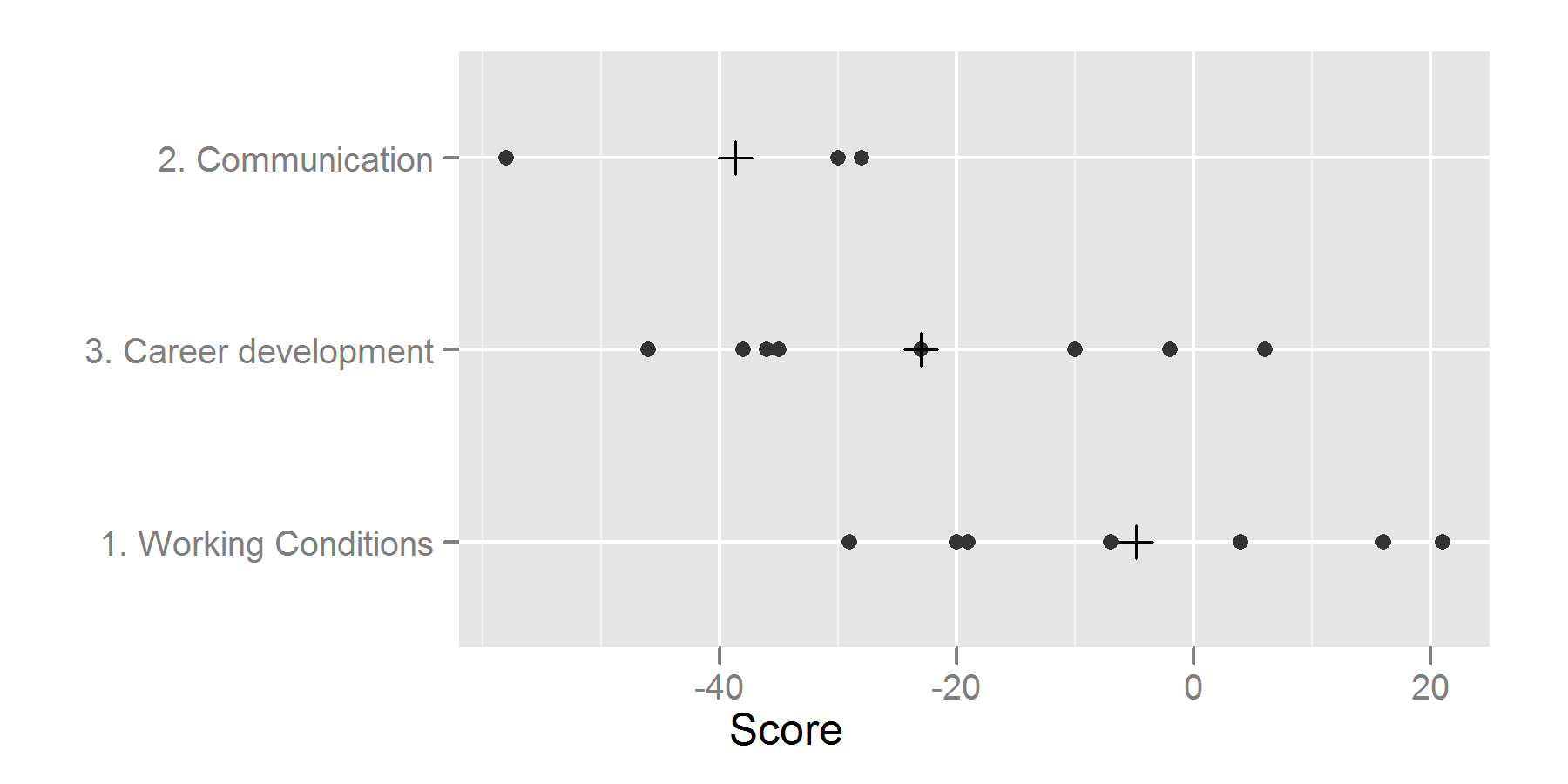Want to share your content on R-bloggers? click here if you have a blog, or here if you don't.

Last week I was shown the results of a workplace happiness questionnaire.  There’s a cut down version of the dataset here, with numbers and wording changed to protect the not-so-innocent.

The plots were ripe for a makeover.  The ones I saw were 2nd hand photocopies, but I’ve tried to recreate their full glory as closely as possible.To the creator’s credit, they have at least picked the correct plot-type: a stacked bar chart is infinitely preferable to a pie chart.  That said, there’s a lot of work to be done.  Most obviously, the pointless 3D effect needs removing, and the colour scheme is badly chosen.  Rainbow style colour schemes that change hues are best suited to unordered categorical variables.  If you have some sense of ordering to the variable then a sequential scale is more appropriate.  That means keeping the hue fixed and either scaling from light to dark, or from grey to a saturated colour.  In this case, we have ordering and also a midpoint – the “neutral” response.  That means that we should use a diverging scale (where saturation or brightness increases as you move farther from the mid-point).

More problematic than these style issues is that it isn’t easy to answer any useful question about the dataset.  To me, the obvious questions are

On balance, are people happy?

Which questions indicate the biggest problems?

Which sections indicate the biggest problems?

All these questions  require us to condense the seven points of data for each question down to a single score, so that we can order the questions from most negative to most positive.  The simplest, most obvious scoring system is a linear one.  We give -3 points for “strongly disagree”, -2 for “disagree”, through to +3 for “strongly agree”.  (In this case, all the questions are phrased so that agreeing is a good thing.  A well designed questionnaire should contain a balance of positively and negatively phrased questions to avoid yes ladder (link is NSFW) type effects.  If you have negatively phrased questions, you’ll need to reverse the scores.  Also notice that each question uses the same multiple choice scale.  If your questions have different numbers of responses, or more than one answer is allowed then it may be inappropriate to compare the questions.)

Since the scoring system is slightly arbitrary, it is best practise to check your results under a different scoring system.  Perhaps you think that the “strongly” responses should be more heavily weighted, in which case a quadratic scoring system would be appropriate.  (Replace the weights 1:3 with (1:3)^2/2.)  Assume the data is stored in the data frame `dfr`.

```dfr\$score.linear <- with(dfr,
-3 * strongly.disagree - 2 * disagree - slightly.disagree +
slightly.agree + 2 * agree + 3 * strongly.agree)
-4.5 * strongly.disagree - 2 * disagree - 0.5 * slightly.disagree +
0.5 * slightly.agree + 2 * agree + 4.5 * strongly.agree)
```

For the rest of this post, I’ll just present results and code for the linear scoring system. Switch the word “linear” with “quad” to see the alternative results. To get an ordering from “worst” to “best”, we order by `-score.linear`.

```dfr_linear <- within(dfr,
{
question <- reorder(question, -score.linear)
section <- reorder(section, -score.linear)
})
```

To make the data frame suitable for plotting with ggplot, we reshape it from wide to long format.

```library(reshape)
w2l <- function(dfr) melt(dfr, measure.vars = colnames(dfr)[4:10])
mdfr_linear <- w2l(dfr_linear)
```

To answer the first question, we simply take a histogram of the score, and see if they are mostly above or below zero.

```library(ggplot2)
hist_scores_linear <- ggplot(dfr, aes(score.linear)) + geom_histogram(binwidth = 10)
```Hmm, not good. Most of the questions had a negative score, implying that the workforce seems unhappy. Now we want to know why they are unhappy. Here are the cleaned up versions of those stacked bar charts again. As well as the style improvements mentioned above, we plot all the questions together, and in order of increasing score (so the problem questions are the first things you read).

```bar_all_q_linear <- ggplot(mdfr_linear, aes(question, value, fill = variable)) +
geom_bar(position = "stack") +
coord_flip() +
xlab("") +
ylab("Number of responses") +
scale_fill_brewer(type = "div")
```So deaf managers are the biggest issue.  Finally, it can be useful to know if which of the sections scored badly, to find more general problem areas. First we find the mean score by section.

```mean_by_section <- with(dfr_linear, tapply(score.linear, section, mean))
dfr_mean_by_section <- data.frame(
value = mean_by_section,
section = names(mean_by_section)
)
```

Now we visualise these scores as a dotplot.

```plot_by_section <- function(p)
{
p + geom_point(colour = "grey20") +
geom_point(aes(value, section),
data = dfr_mean_by_section,
xlab("Score") + ylab("")
}

pt_by_section_linear <- plot_by_section(
ggplot(mdfr_linear, aes(score.linear, section))
)
```Here you can see that communication the biggest problem area.

Tagged: data-viz, r